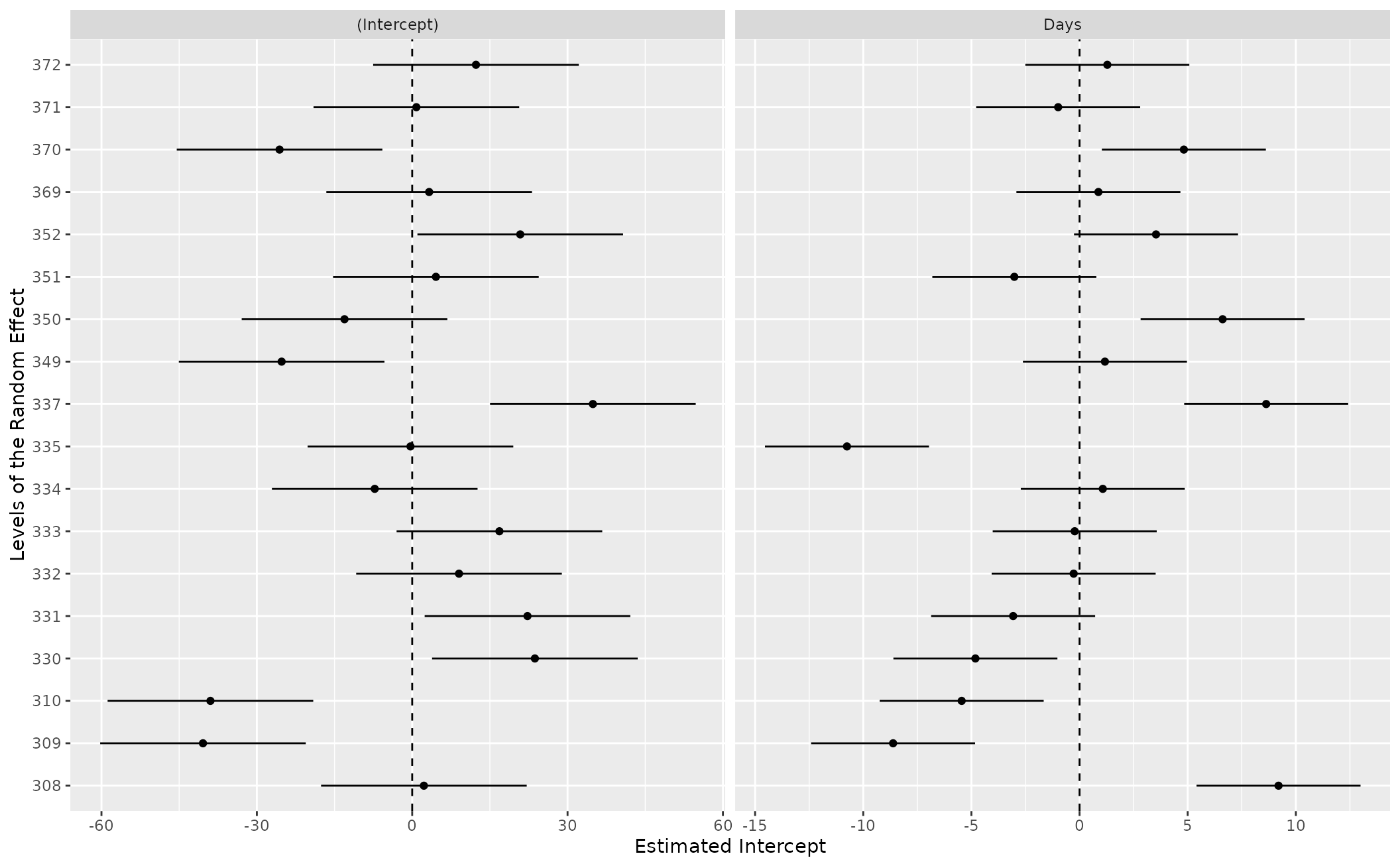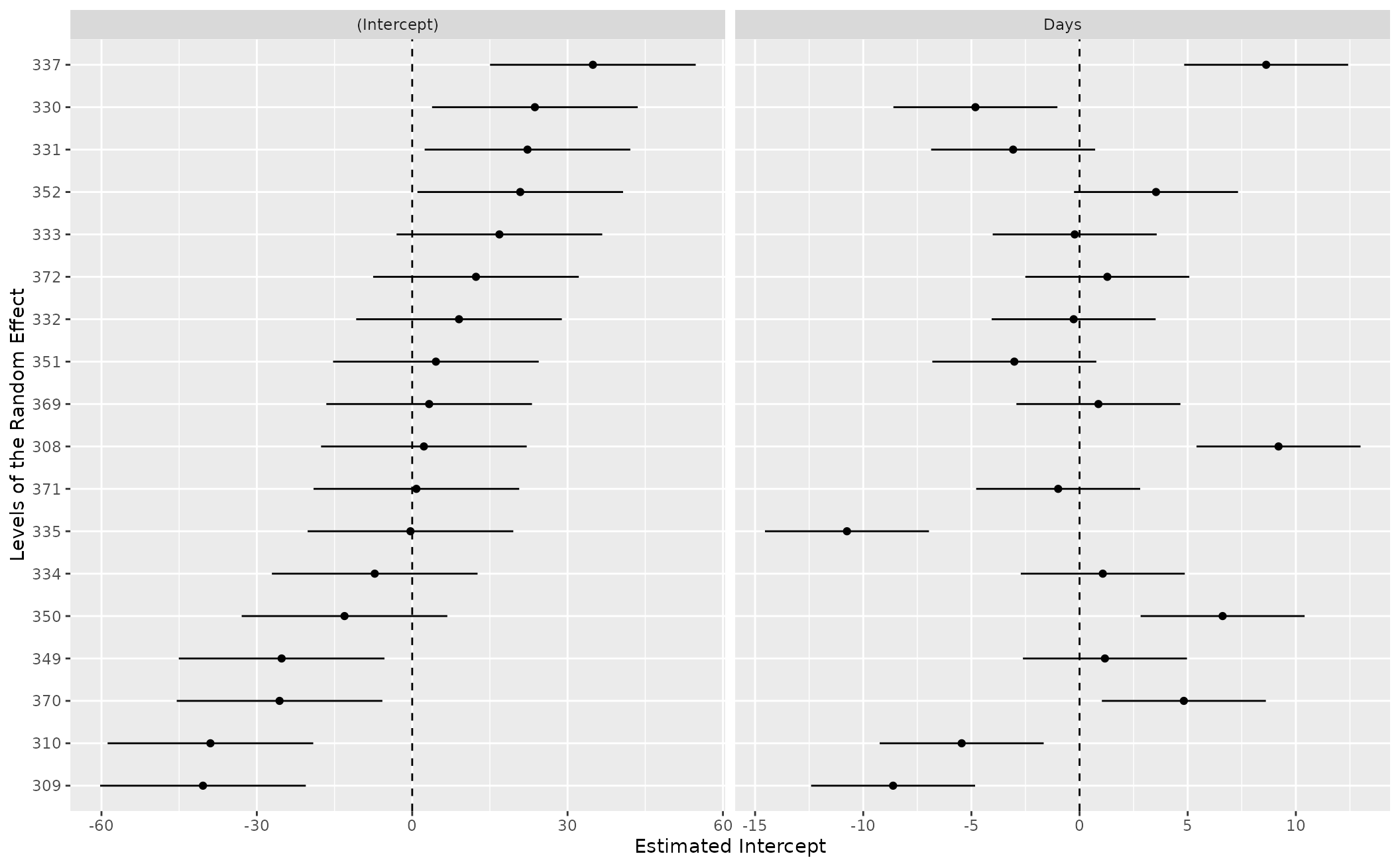show_ranef() allows a user estimating a mixed model to quickly plot the random intercepts (with conditional variances) of a given random effect in a mixed model. In cases where there is a random slope over the intercept, the function plots the random slope as another caterpillar plot (as another facet)

show_ranef(data, grp, reorder = TRUE)

## Arguments

data a fitted mixed model with random intercepts What random intercept/slopes do you want to see as a caterpillar plot? Declare it as a character optional argument. DEFAULT is TRUE, which re-orders'' the intercepts by the original value in the data. If FALSE, the ensuing caterpillar plot defaults to a default method of ordering the levels of the random effect by their estimated conditional mode.

## Value

show_ranef() returns a caterpillar plot of the random intercepts from a given mixed model. If broom.mixed::augment() can process it, this function should work just fine.

## Details

This function is a simple wrapper in which broom.mixed and, obviously ggplot2 are doing the heavy lifting.

Steven V. Miller

## Examples


library(lme4)
library(stevemisc)
data(sleepstudy)

M1 <- lmer(Reaction ~ Days + (Days | Subject), data=sleepstudy)
show_ranef(M1, "Subject")show_ranef(M1, "Subject", reorder=FALSE)